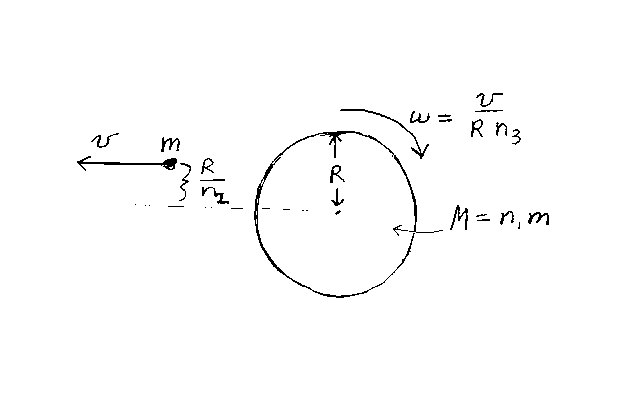Problem D12: A child of mass m is sitting on a cylindrical merry-go-round, which has a mass of n1m. The radius of the merry-go-round is R. Initially, the child is sitting a distance R/n2 from the center, and both child and merry-go-round are at rest. Then, the child jumps off in a direction perpendicular to the radius. If the speed of the child with respect to the gound is v, what is the final angular velocity ω of the merry-go-round? If ω=v/(R n3) What is n3? Note that n1, n2, and n3 are unitless. Assume that the merry-go-round is a cylindrical disk.n1 = n2 = Input n3:
If you are currently in my class, you can record your grade by entering your name and student ID number (without the leading zeros) below and clicking on "record grade".
 First Name = Last Name = ID = Problem: